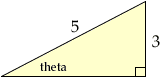Subject: Trigonometry Name: Jason Parks Who is asking: Student Level: Secondary Question: Find value. Assume that all angles are in Quadrant 1. cot(arcsin 3/5) Thanks Hi Jason, Suppose that arcsin(3/5) = theta, that is theta is the angle whose sine is 3/5. Since the angles are all in the first quadrant you can draw a representative right triangle.Use the theorem of pythagoras to find the third side of the triangle and then you can read the cotangent of theta off the diagram. Cheers, Harley Go to Math Central